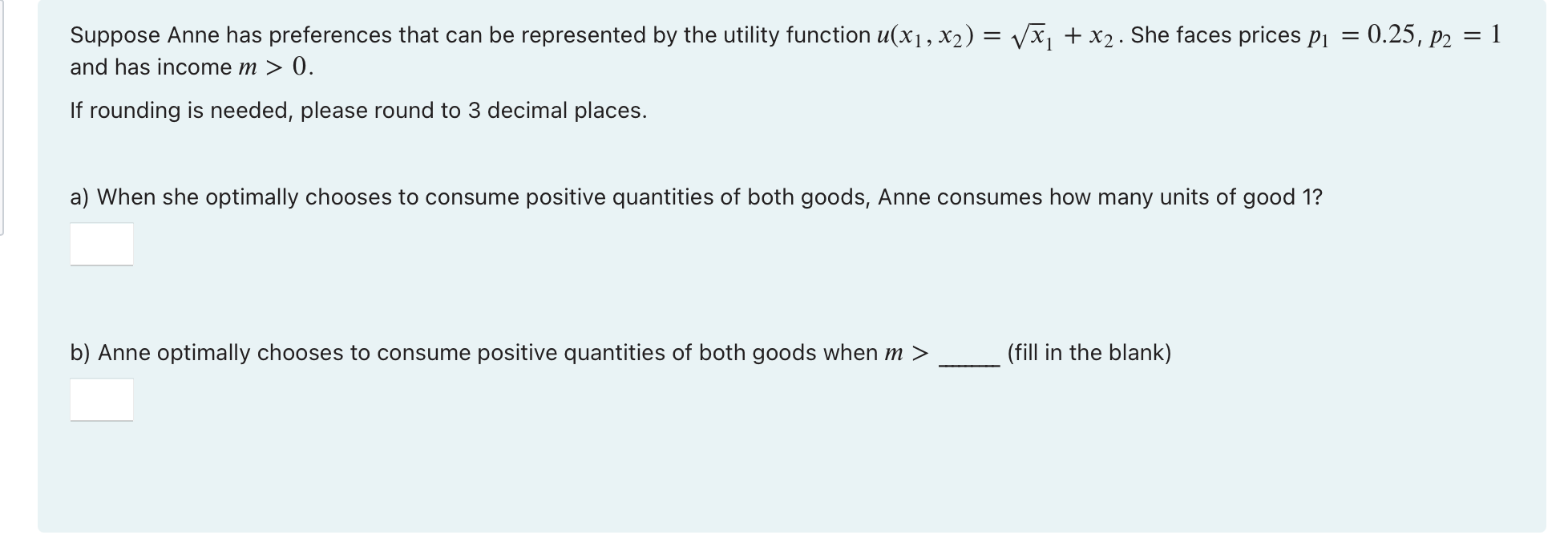### Create an Account

Already have account?

### Forgot Your Password ?

Home / Questions / Suppose Anne has preferences that can be represented by the utility function u(x1, x2) = V...

# Suppose Anne has preferences that can be represented by the utility function u(x1, x2) = Vx1 + x2. She faces prices P1 = 0.25, P2 = 1 and has income m > 0. If rounding is needed, please round to

Suppose Anne has preferences that can be represented by the utility function u(x1, x2) = Vx1 + x2. She faces prices P1 = 0.25, P2 = 1 and has income m > 0. If rounding is needed, please round to 3 decimal places. a) When she optimally chooses to consume positive quantities of both goods, Anne consumes how many units of good 1? b) Anne optimally chooses to consume positive quantities of both goods when m > (fill in the blank)Jun 05 2021 View more View Less

#### Answer (Solved)Subscribe To Get Solution